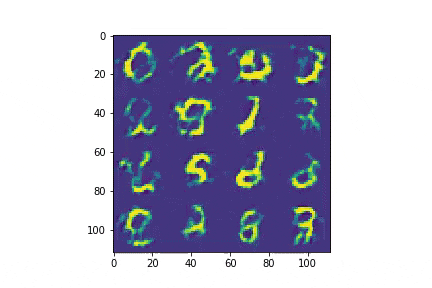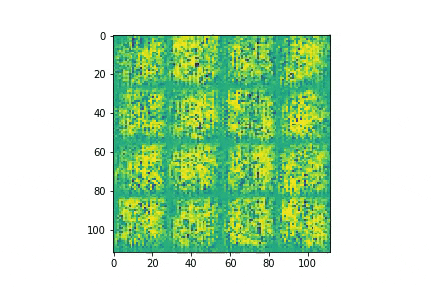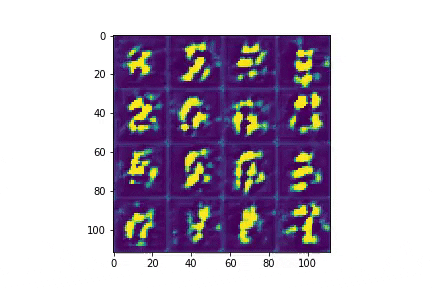GANs 3/nAh, WGANs — the sophisticated choice, the thinking man's GAN. Unfortunately, I wasn't really able to get them to work, revealing my bourgeois-GAN origins. Still, there were things to learn — I'll jump to that first, leaving the setup info at the end. Because I was never able to get this WGAN to work well, it's possible that I've made deeper mistakes below the survace; I'll try to call out some hints about that too.

Things I learned: Weight Clipping Matters

Famously, you're supposed to clip the discriminator's weights for a WGAN. This is a crude way to get a convergence result to hold by forcing D to be K-Lipschitz; my understanding is there are more sophisticated regularization schemes that work better, but that's a topic for another blog post. For now, the relevant part of code is in the discriminator's training loop, where we've got a snippet that looks like this:

for p in self.D.parameters():
p.data.clamp_(-0.04, 0.04)

Why -0.04 to 0.04, you ask? I don't have a satisfying answer — this is trial and error. 0.01 doesn't work well, and neither does 0.1, but 0.04 seems to do ok. Intuitively, it seems like clipping too narrowly would force the discriminator into a suboptimal solution (at the edge of the clip hypercube). Maybe clipping too widely leads to a vast solution space, and convergence takes too long for me to observe it in practice. These are just-so speculations, though, and I really don't know why, in this specific case, 0.04 works, but 0.1 and 0.01 do not.

It's interesting what failure looks like. Here's what happens with a weight clip of 0.1 (showing 10 epochs only):(Yes, that really is a gif; it's just not changing much.) And here's what happens with a weight clip of 0.01 (again, first 10 epochs only):It's interesting what happens to D's mean scores too. With a weight clip of 0.1, D quickly learns to map almost all real images to 1 and almost all fake images to 0. With a weight clip of 0.01, D gives a 50/50 guess for each.

I'll end this section by quoting the relevant part of the paper, which suggests similar issues:

If the clipping parameter is large, then it can take a long time for any weights to reach their limit, thereby making it harder to train the critic till optimality. If the clipping is small, this can easily lead to vanishing gradients when the number of layers is big, or batch normalization is not used (such as in RNNs).

Training Setup

Here's the setup that I used in the end. The network for the generator looks like this:

class G(nn.Module):

def __init__(self):
super().__init__()
self.conv_layers = nn.Sequential(
nn.ConvTranspose2d(100, 64, 5, 2, 0),
nn.BatchNorm2d(64),
nn.ReLU(True),
nn.ConvTranspose2d( 64, 16, 4, 2, 0),
nn.BatchNorm2d(16),
nn.ReLU(True),
nn.ConvTranspose2d( 16,  4, 4, 2, 0),
nn.BatchNorm2d(4),
nn.ReLU(True),
nn.ConvTranspose2d(  4,  1, 3, 1, 0),
nn.Tanh()
)

def forward(self, z):
return self.conv_layers(z)

(This is exactly the same as the DCGAN of the last post.) And similarly for the discriminator:

class D(nn.Module):

def __init__(self):
super().__init__()
self.conv_layers = nn.Sequential(
nn.Conv2d(1, 4, 3, 1, 0),
nn.BatchNorm2d(4),
nn.LeakyReLU(0.2, inplace=True),
nn.Conv2d(4, 16, 4, 2, 0),
nn.BatchNorm2d(16),
nn.LeakyReLU(0.2, inplace=True),
nn.Conv2d(16, 64, 4, 2, 0),
nn.BatchNorm2d(64),
nn.LeakyReLU(0.2, inplace=True),
nn.Conv2d(64, 256, 5, 2, 0),
)
self.linear_layers = nn.Sequential(
nn.Linear(16*16, 16),
nn.Linear(16, 1),
nn.Sigmoid()
)

def forward(self, x):
bs = x.shape
y1 = self.conv_layers(x)
y2 = self.linear_layers(y1.view(bs, -1))
return y2

Training WGANs is a little more complicated. Here's what G's training loop looks like:

def train_G(self):

# Don't accumulate gradients for D while training G.
for p in self.D.parameters():

# Train G so that D labels G's fake images as real.
z = Variable(
torch.randn(self.data_loader.batch_size, 100, 1, 1)
).to(self.device)
fake_images = self.G(z)
D_on_fake = self.D(fake_images).view(-1) # This is why we need D's grad off.
loss = -1*D_on_fake.mean(0).view(1)
loss.backward()
self.G_opt.step()

# Turn D's gradient accumulation back on.
for p in self.D.parameters():

return { 'G_loss': loss.detach().cpu().numpy() }

The thing to point out is that G's loss function is simply -1 times the average score of D on fake images (the ones generated by G). Similarly for D's training loop:

def train_D(self, image_batch):

for p in self.D.parameters():
p.data.clamp_(-0.04, 0.04)

real_images = Variable(image_batch).to(self.device)

# Train D to map real images to 1.
D_on_real = self.D(real_images).view(-1)
D_loss_on_real = D_on_real.mean(0).view(1)

# Train D to map fake (generated) images to 0.
z = Variable(
torch.randn(self.data_loader.batch_size, 100, 1, 1)
).to(self.device)
with torch.no_grad(): # Don't accumulate gradients for G while training D
D_on_fake = self.D(fake_images).view(-1)
D_loss_on_fake = D_on_fake.mean(0).view(1)

D_loss = D_loss_on_fake - D_loss_on_real
D_loss.backward()
self.D_opt.step()

return {
'D_loss_on_real': D_loss_on_real.detach().cpu().numpy(),
'D_loss_on_fake': D_loss_on_fake.detach().cpu().numpy(),
'D_loss'        : D_loss.detach().cpu().numpy(),
'D_on_real_sum' : D_on_real.detach().cpu().numpy().sum(),
'D_on_fake_sum' : D_on_fake.detach().cpu().numpy().sum(),
}

Notice that the loss for D is D_loss_on_fake - D_loss_on_real. We're minimizing this, so we want D to map fake images to low numbers and real images to high numbers. The world makes sense. The only other thing of interest to note is that in the loop itself, we train D twice as much as we train G — this is a lazy attempt to get closer to the WGAN paper's ideal, which is for D to be perfectly trained in each epoch before G is.

I used the Adam optimizer for both G and D (betas of (0.5, 0.999), learning rate of 4e-4), and optimized against BCELoss. I trained a Colab notebook.

Results

As above, here's what the results look like after 50 epochs:I don't think these look great. They're clearly optimized for something, but to my eye they look worse than the ones from the simple DCGAN.

Here are the losses by epoch:And here are the mean discriminator scores (0 for fake, 1 for real):